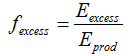# HOMER Pro 3.11

 Navigation: Glossary Excess Electricity FractionType: Output Variable Units: none Symbol: fexcess

The excess electricity fraction is the ratio of total excess electricity to the total electrical production. HOMER calculates this value at the end of each simulation using the following equation:where: Eexcess [kWh/yr] Eprod = total electrical production [kWh/yr]

See also

Total Excess Electricity